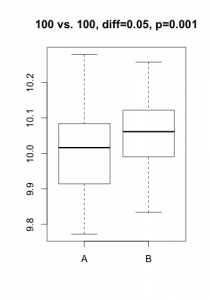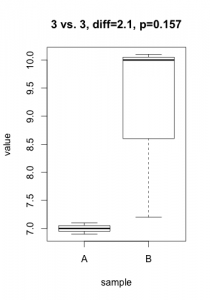Much has been written on the need for statistics in genome-scale molecular biology.  Very clever analytical approaches were devised, taking the form of carefully crafted and freely downloadable software packages.  But still, every month or so, I meet with students and researchers facing a similar dilemma:  they need to decide whether to report the strength of an effect (eg. gene X is over-expressed by 4.5-fold in condition A vs. B) or the significance of such effect (eg. gene X is overexpressed in condition A vs. B with a p-value of 0.0012).

A p-value is always linked to a statistical test which is nothing more than a mathematical formulation of a very precise question on the data.  To derive a p-value, this question takes the form of an hypothesis (eg. gene X is not differentially expressed), called $H_0$ (null hypothesis) in the statistical jargon.  A low p-value suggests that the hypothesis doesn’t hold (eg. the data does not support that gene X is not differentially expressed…  notice the double negation!).  In practice, this nuance bites in two cases: tiny, irrelevant differences in large datasets or solid differences accompanied with unexpected noise.  Here are two simulated datasets (imagine qPCR quantification of a gene for samples under two conditions).In the first scenario, the statistical test comes out significant even if the actual difference in expression is probably irrelevant (3% increase) and probably the result of some unwanted bias.  In the second scenario, one of the 3 samples under condition ‘B’ misbehaved, resulting in a large difference becoming non-significant.  For both cases, the average difference in expression would give a much better indication of what is going on.  In most projects I’ve been involved in (microarrays, RNA-Seq, HTS, proteomics, qPCR, etc.), my first suggestion is always to start analyzing the results using strength of effect (differences, log-fold-change, %inhibition, etc.).

Then, what is the point of applying statistical tests? In what cases does it actually serve the underlying scientific question (does conditions A or B impact expression of gene X)?  I’ll come back to this in a later post…  but feel free to share your opinion in the meantime!